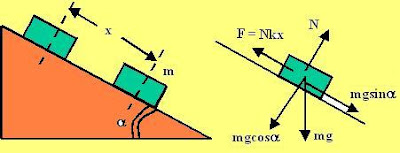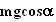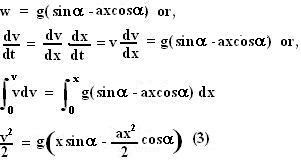## Saturday, November 24, 2007

### Irodov Problem 1.102Let the mass of the body be m and let the force of friction acting on it be F. F will be proportional to N and be equal to Nax. The forces acting on the body are shown in the Figure. We can resolve all the forces along parallel and perpendicular direction to the slope.

Along the Perpendicular Direction there are two forces, i) the Normal reaction from the surface N and ii) the component of gravityand so we have,Along the Parallel Direction there are two forces acting, i) the component of gravityand ii) the force of friction Nkx. Let the acceleration of the mass be w. So we have,From (1) and (2) we have,When the mass finally comes to stop v=0, putting this in (3) we have.

The maximum velocity occurs when acceleration has become 0 (w=0) and is about to reverse its direction. This occurs when. Substituting the value in (3) we have the maximum velocity as.